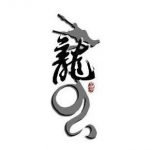• 欢迎访问少将全栈，学会感恩，乐于付出，珍惜缘份，成就彼此、推荐使用最新版火狐浏览器和Chrome浏览器访问本网站。
• 吐槽，投稿，删稿，交个朋友，商务沟通欢迎加博主微信：amlhbmdfc2hhb2Jv (Base64) 接头暗号：少将
• 如果您觉得本站非常有看点，那么赶紧使用Ctrl+D 收藏少将全栈吧
• 欢迎加博主微信：amlhbmdfc2hhb2Jv (Base64) 接头暗号：少将

# python 变量赋值 图解

8年前 (2015-04-16) 2377次浏览

``a = 1 ``

``t_007 = ’T007’ ``

``Answer = True ``

Python中，等号`=`是赋值语句，可以把任意数据类型赋值给变量，同一个变量可以反复赋值，而且可以是不同类型的变量，例如：

``````a = 123 # a是整数
print a
a = ’ABC’ # a变为字符串
print a ``````

``````int a = 123; // a是整数类型变量
a = "ABC"; // 错误：不能把字符串赋给整型变量 ``````

``````x = 10
x = x + 2 ``````

``a = ’ABC’ ``

1. 在内存中创建了一个`’ABC’`的字符串；

2. 在内存中创建了一个名为`a`的变量，并把它指向`’ABC’`

``````a = ’ABC’
b = a
a = ’XYZ’
print b ``````

[🍬谢谢你请我吃糖果🍬🍬～]